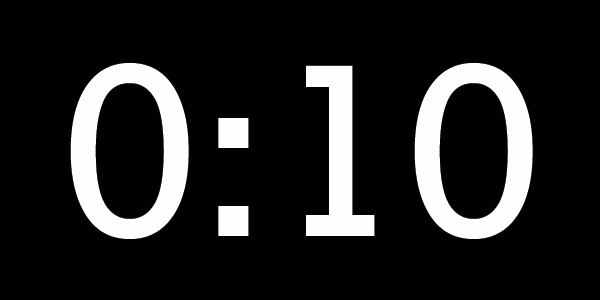# Countdown

Logic Level 1$\huge 3 ~ \square ~ 3 ~ \square ~ 3 ~ \square ~ 3= 0$

Fill the three squares with any of the operators $+,-,\times, \div$. Use one operator exactly once.

Can we make the equation true?

×